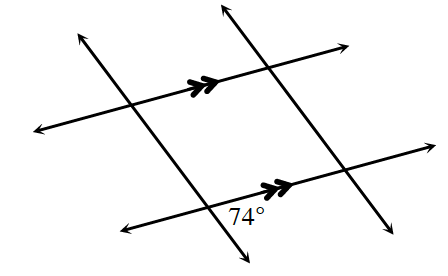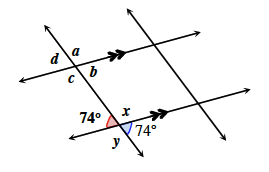### Home > GB8I > Chapter 2 Unit 2 > Lesson INT1: 2.1.1 > Problem2-10

2-10.

Examine the diagram at right. Based on the information in the diagram, which angles can you determine the measures of? Copy the diagram on your paper and determine the measures of only those angles that you can justify.Refer to the Math Notes box in Lesson 1.2.1 or problem 1-42.

The symbols on two of the lines indicate that those lines are parallel.

The angles marked in the diagram at right are vertical angles, therefore they are congruent.The value of $x$ can be found because it is supplementary with both $74º$ angles.

You can also determine the values of angles a through $d$ since they correspond to the angles you have already determined.